# Java 2

26. Jul 2012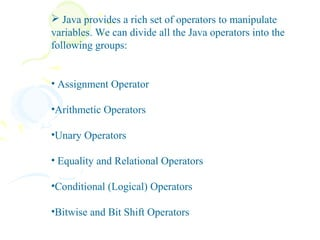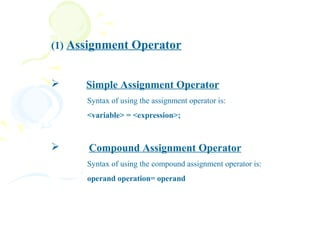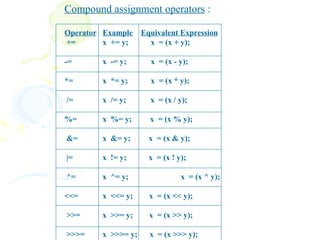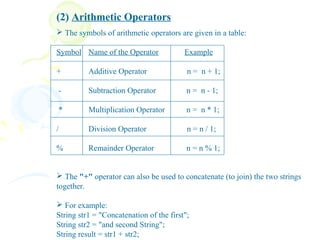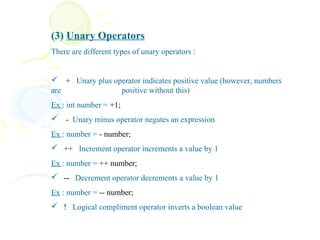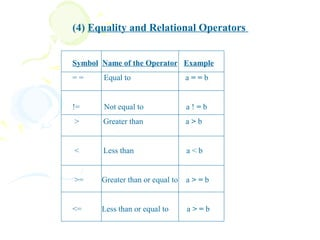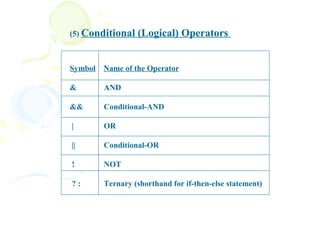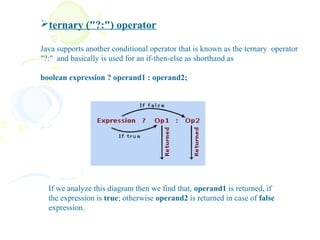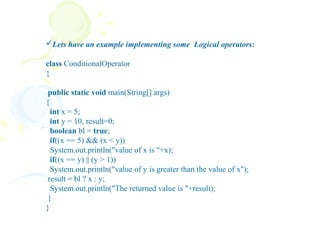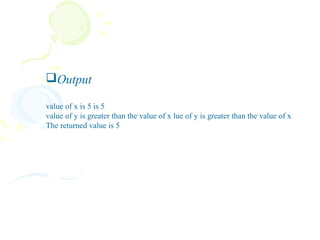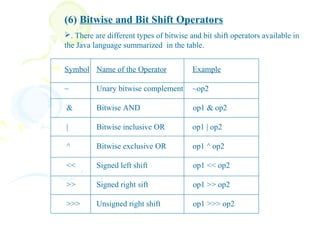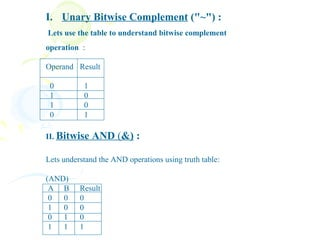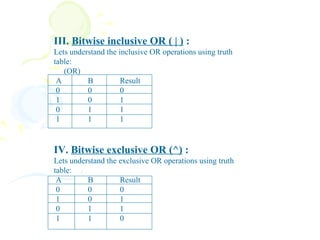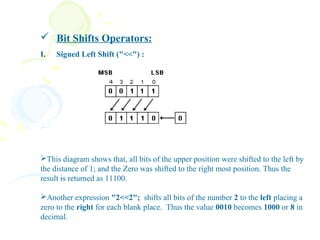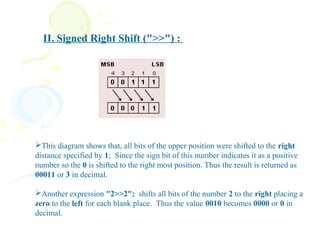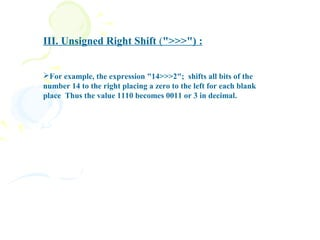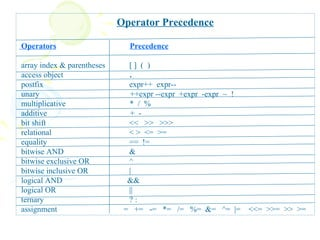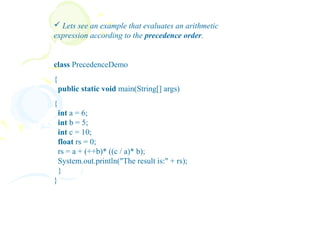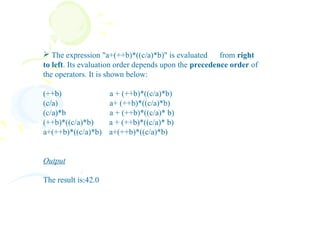1 von 21

### Java 2

• 2.  Java provides a rich set of operators to manipulate variables. We can divide all the Java operators into the following groups: • Assignment Operator •Arithmetic Operators •Unary Operators • Equality and Relational Operators •Conditional (Logical) Operators •Bitwise and Bit Shift Operators
• 3. (1) Assignment Operator  Simple Assignment Operator Syntax of using the assignment operator is: <variable> = <expression>;  Compound Assignment Operator Syntax of using the compound assignment operator is: operand operation= operand
• 4. Compound assignment operators : Operator Example Equivalent Expression += x += y; x = (x + y); -= x -= y; x = (x - y); *= x *= y; x = (x * y); /= x /= y; x = (x / y); %= x %= y; x = (x % y); &= x &= y; x = (x & y); |= x != y; x = (x ! y); ^= x ^= y; x = (x ^ y); <<= x <<= y; x = (x << y); >>= x >>= y; x = (x >> y); >>>= x >>>= y; x = (x >>> y);
• 5. (2) Arithmetic Operators  The symbols of arithmetic operators are given in a table: Symbol Name of the Operator Example + Additive Operator n = n + 1; - Subtraction Operator n = n - 1; * Multiplication Operator n = n * 1; / Division Operator n = n / 1; % Remainder Operator n = n % 1;  The "+" operator can also be used to concatenate (to join) the two strings together.  For example: String str1 = "Concatenation of the first"; String str2 = "and second String"; String result = str1 + str2;
• 6. (3) Unary Operators There are different types of unary operators :  + Unary plus operator indicates positive value (however, numbers are positive without this) Ex : int number = +1;  - Unary minus operator negates an expression Ex : number = - number;  ++ Increment operator increments a value by 1 Ex : number = ++ number;  -- Decrement operator decrements a value by 1 Ex : number = -- number;  ! Logical compliment operator inverts a boolean value
• 7. (4) Equality and Relational Operators Symbol Name of the Operator Example == Equal to a==b != Not equal to a!=b > Greater than a>b < Less than a<b >= Greater than or equal to a>=b <= Less than or equal to a>=b
• 8. (5) Conditional (Logical) Operators Symbol Name of the Operator & AND && Conditional-AND | OR || Conditional-OR ! NOT ?: Ternary (shorthand for if-then-else statement)
• 9. ternary ("?:") operator Java supports another conditional operator that is known as the ternary operator "?:" and basically is used for an if-then-else as shorthand as boolean expression ? operand1 : operand2; If we analyze this diagram then we find that, operand1 is returned, if the expression is true; otherwise operand2 is returned in case of false expression.
• 10. Lets have an example implementing some Logical operators: class ConditionalOperator { public static void main(String[] args) { int x = 5; int y = 10, result=0; boolean bl = true; if((x == 5) && (x < y)) System.out.println("value of x is "+x); if((x == y) || (y > 1)) System.out.println("value of y is greater than the value of x"); result = bl ? x : y; System.out.println("The returned value is "+result); } }
• 11. Output value of x is 5 is 5 value of y is greater than the value of x lue of y is greater than the value of x The returned value is 5
• 12. (6) Bitwise and Bit Shift Operators . There are different types of bitwise and bit shift operators available in the Java language summarized in the table. Symbol Name of the Operator Example ~ Unary bitwise complement ~op2 & Bitwise AND op1 & op2 | Bitwise inclusive OR op1 | op2 ^ Bitwise exclusive OR op1 ^ op2 << Signed left shift op1 << op2 >> Signed right sift op1 >> op2 >>> Unsigned right shift op1 >>> op2
• 13. I. Unary Bitwise Complement ("~") : Lets use the table to understand bitwise complement operation : Operand Result 0 1 1 0 1 0 0 1 II. Bitwise AND (&) : Lets understand the AND operations using truth table: (AND) A B Result 0 0 0 1 0 0 0 1 0 1 1 1
• 14. III. Bitwise inclusive OR ( | ) : Lets understand the inclusive OR operations using truth table: (OR) A B Result 0 0 0 1 0 1 0 1 1 1 1 1 IV. Bitwise exclusive OR (^) : Lets understand the exclusive OR operations using truth table: A B Result 0 0 0 1 0 1 0 1 1 1 1 0
• 15.  Bit Shifts Operators: I. Signed Left Shift ("<<") : This diagram shows that, all bits of the upper position were shifted to the left by the distance of 1; and the Zero was shifted to the right most position. Thus the result is returned as 11100. Another expression "2<<2"; shifts all bits of the number 2 to the left placing a zero to the right for each blank place. Thus the value 0010 becomes 1000 or 8 in decimal.
• 16. II. Signed Right Shift (">>") : This diagram shows that, all bits of the upper position were shifted to the right distance specified by 1; Since the sign bit of this number indicates it as a positive number so the 0 is shifted to the right most position. Thus the result is returned as 00011 or 3 in decimal. Another expression "2>>2"; shifts all bits of the number 2 to the right placing a zero to the left for each blank place. Thus the value 0010 becomes 0000 or 0 in decimal.
• 17. III. Unsigned Right Shift (">>>") : For example, the expression "14>>>2"; shifts all bits of the number 14 to the right placing a zero to the left for each blank place Thus the value 1110 becomes 0011 or 3 in decimal.
• 18. Operator Precedence Operators Precedence array index & parentheses [] ( ) access object . postfix expr++ expr-- unary ++expr --expr +expr -expr ~ ! multiplicative * / % additive + - bit shift << >> >>> relational < > <= >= equality == != bitwise AND & bitwise exclusive OR ^ bitwise inclusive OR | logical AND && logical OR || ternary ?: assignment = += -= *= /= %= &= ^= |= <<= >>= >> >=
• 19.  Lets see an example that evaluates an arithmetic expression according to the precedence order. class PrecedenceDemo { public static void main(String[] args) { int a = 6; int b = 5; int c = 10; float rs = 0; rs = a + (++b)* ((c / a)* b); System.out.println("The result is:" + rs); } }
• 20.  The expression "a+(++b)*((c/a)*b)" is evaluated from right to left. Its evaluation order depends upon the precedence order of the operators. It is shown below: (++b) a + (++b)*((c/a)*b) (c/a) a+ (++b)*((c/a)*b) (c/a)*b a + (++b)*((c/a)* b) (++b)*((c/a)*b) a + (++b)*((c/a)* b) a+(++b)*((c/a)*b) a+(++b)*((c/a)*b) Output The result is:42.0